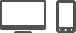# Custom types and WinJS.ClassYou can use the WinJS.Class.define and WinJS.Class.derive functions to define and derive JavaScript types.

## Defining classes with WinJS.Class.define

The WinJS.Class.define function is a helper function for defining a JavaScript type. You supply a constructor function, a set of "instance" members (which are defined on the prototype of the type), and a set of "static" members (which are defined on the type itself).

The properties that are added to the prototype of an object appear on any object that is instantiated by calling its constructor function, but the properties added to the type directly belong only to the type itself. For example, the following code shows how to define a type `Robot` that has an instance property `name` and a static property `harmsHumans`.

The `modelName` property belongs to the individual objects that are created with the `Robot(name)` constructor function, but the `harmsHumans` property is valid for all `Robot` objects.

``````var Robot = WinJS.Class.define(
// The constructor function.
function(name) {
this.name = name;
},
// The set of instance members.
{ modelName: "" },
// The set of static members.
{ harmsHumans: false });

var myRobot = new Robot("Mickey");

myRobot.modelName = "4500";
Robot.harmsHumans = false;
``````

The code above corresponds directly to the following "straight" JavaScript code:

``````function Robot(name) {
this.name = name;
this.modelName = "";
}

var myRobot = new Robot("Mickey")

myRobot.modelName = '4500';
Robot.harmsHumans = false;
``````

WinJS.Class.define offers helpful shortcuts for defining properties. In JavaScript you can customize the behavior of properties by using a property descriptor. WinJS.Class.define treats a given object as a property descriptor. For example, if you want to use a computed value for `modelName`, in JavaScript without Windows Library for JavaScript you need the following code:

``````Object.defineProperty(Robot.prototype, "modelName", {
get: function () { this.computeModelName(); }
});
``````

When you use WinJS.Class.define, you can write code like the following.

``````var Robot = WinJS.Class.define(
function (name) { this.name = name; },
{
modelName: { get: function () { return this.computeModelName(); } }
}
);
``````

## Deriving classes with WinJS.Class.derive

The WinJS.Class.derive function is a helper function that you can use to derive one type from another. It behaves much like WinJS.Class.define, except that it uses the prototype of the base type to construct the derived type by calling Object.create. The Object.create method can be used to derive one type from another by using the prototype of the parent object, plus any properties that are added to the child object. Both WinJS.Class.derive and Object.create create a base type (that is not derived from any other object) if the first parameter is null.

The following code shows how to derive a `SpaceRobot` type from the `Robot` type. You add "static" and "instance" members in the same way as with WinJS.Class.define.

In the following code, the SpaceRobot class is derived from the Robot class. It has all the properties of the Robot class, plus an "instance" property airSupply and a "static" property safeSelf.

``````var SpaceRobot = WinJS.Class.derive(Robot,
// The constructor function.
function (name) {
this.name = name;
},
// The set of instance members.
{ airSupply: "" },
// The set of static members.
{ saveSelf: true });

var mySpaceRobot = new SpaceRobot("Myra");
mySpaceRobot.airSupply = "oxygen";
var save = SpaceRobot.saveSelf;
``````